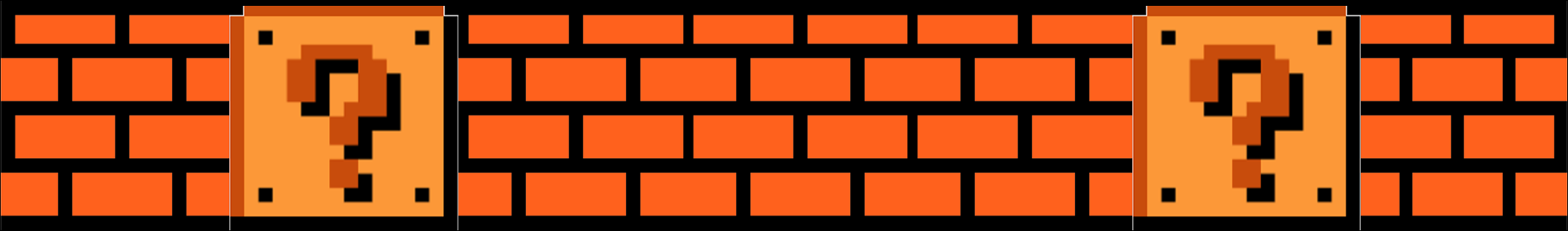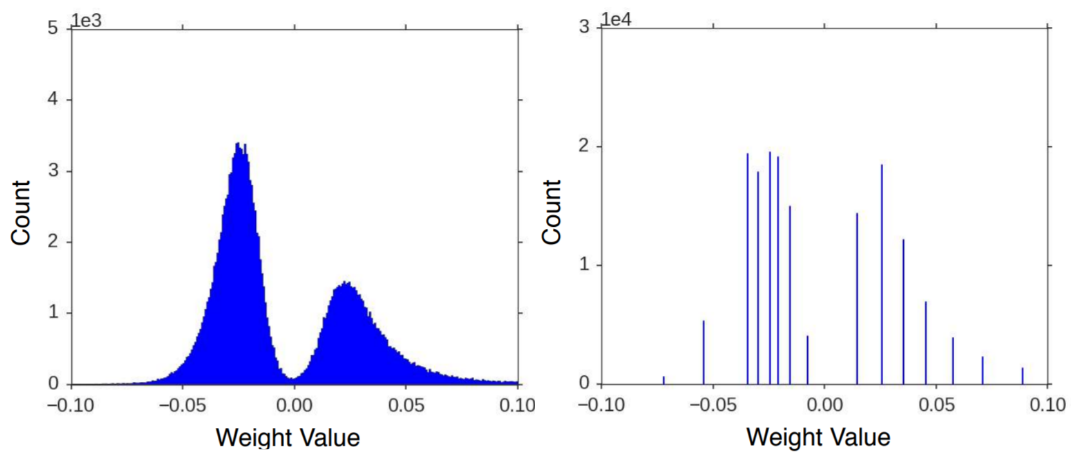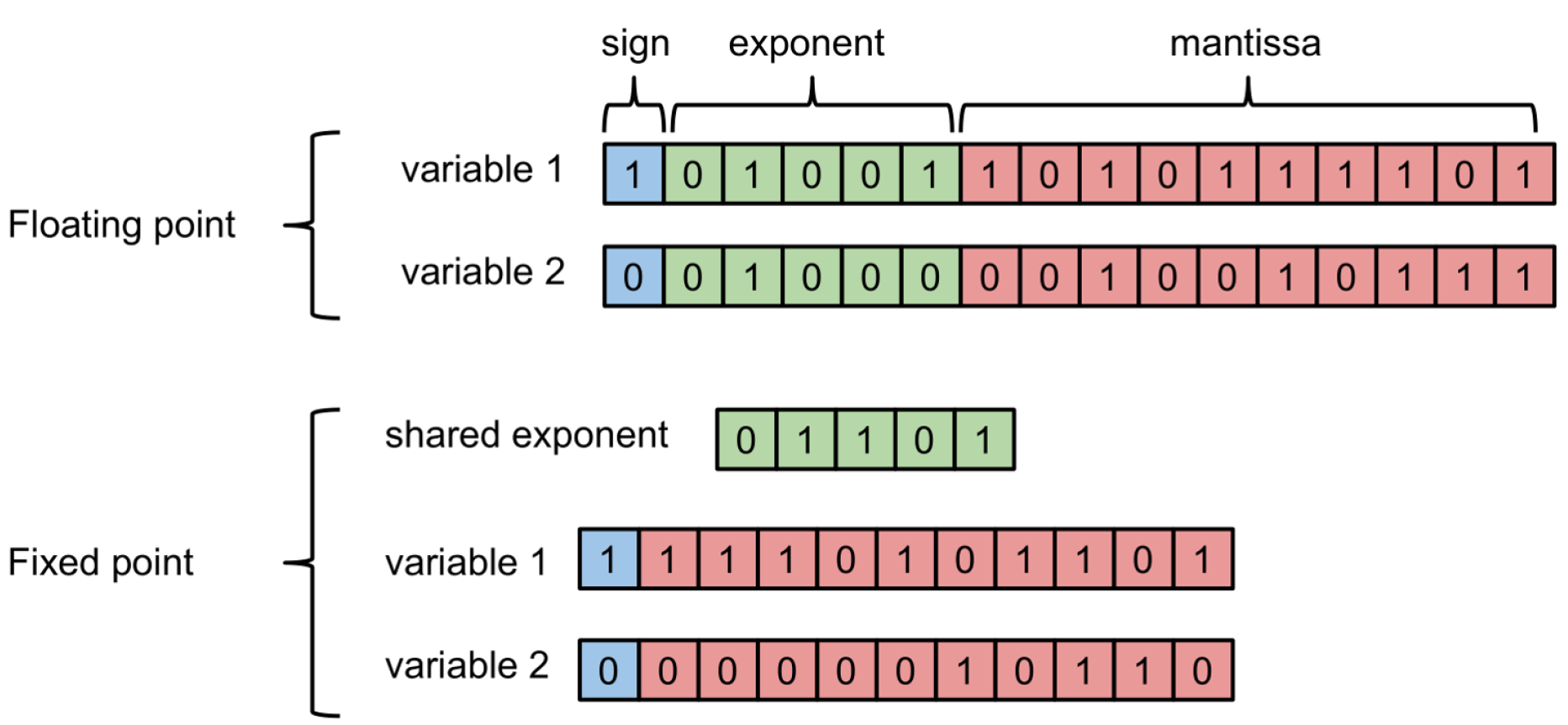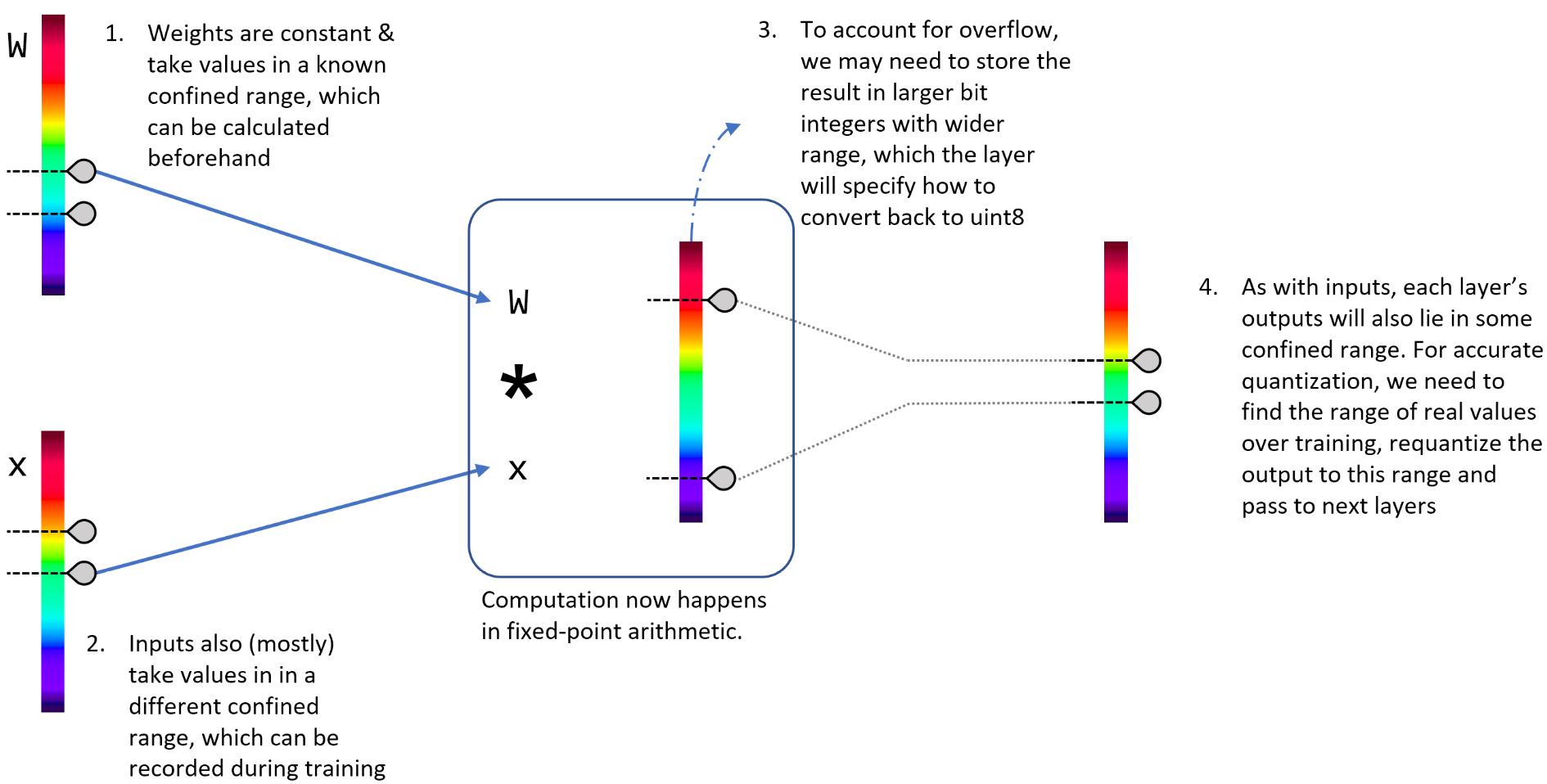# Making Neural Nets Work With Low Precision

8-bit quantization in TensorFlow-Lite

This post was featured on Heartbeat by Fritz and DL Weekly

Francois Chollet puts it concisely:

For many deep learning problems, we’re finally starting with the “make it efficient” stage. We had been stuck at the first two stages for many decades where speed and efficiency weren’t nearly as important as getting things to work in the first place. So, the question of how precise our calculations need to be – and whether we can manage with lower precision – wasn’t often asked. However, now that neural networks are good enough at many problems to be of production-grade or better, this question has risen again. And the answers suggest we could do with low(er) precision, causing what may soon be a paradigm shift in mobile-optimized AI. This post talks about the concept of quantized inference, and how it works in TensorFlow-Lite.

TL;DR just tell me how to quantize my model: Here’s a tutorial from TensorFlow with code

## What is low-precision?

Computers can only use a finite number of bits to represent infinite real numbers. How accurately we can represent them is decided by how many bits we use – with 32-bit floating point being the default for most applications, including deep learning. It turns out that DNNs can work with smaller datatypes, with less precision, such as 8-bit integers. Roughly speaking, we’re trying to work with a number line looking closer to the sparse one on the bottom. The numbers are quantized, i.e. discretized to some specific values, which we can then represent using integers instead of floating-point numbers. To be more precise (no pun), we’ll use 8-bit fixed-point representation, which I’ll get back to in a while.

## Why do we care?

Supporting inference with quantized types in any ML framework like Caffe, TensorFlow, etc. would require us to rework significant parts of the library’s design, as well as re-implement most layers. Yet, there are several reasons that make the gains worth this effort:

• Arithmetic with lower bit-depth is faster, assuming the hardware supports it. Even though floating-point computation is no longer “slower” than integer on modern CPUs, operations with 32-bit floating point will almost always be slower than, say, 8-bit integers.
• In moving from 32-bits to 8-bits, we get (almost) 4x reduction in memory straightaway. Lighter deployment models mean they hog lesser storage space, are easier to share over smaller bandwidths, easier to update, etc.
• Lower bit-widths also mean that we can squeeze more data in the same caches/registers. This means we can reduce how often we access things from RAM, which is usually consumes a lot of time and power.
• Floating point arithmetic is hard – which is why it may not always be supported on microcontrollers on some ultra low-power embedded devices, such as drones, watches, or IoT devices. Integer support, on the other hand, is readily available.

You can see why all of this sounds like great news for someone interested in deep learning applications on mobiles or embedded devices. Deep learning researchers are now finding ways to train models that work better with quantization, ML library developers are building extensive framework support for quantized inference, and tech giants are throwing their weight behind dedicated hardware for AI with emphasis on quantization support ( Google, Huawei, Microsoft, Facebook, Apple… ). Even without such dedicated hardware, DSP chips on modern smartphone chipsets have instruction sets well-suited for this kind of integer computation.

## Why does it work?

There has been an increasing amount of work in quantizing neural networks, and they broadly point to two reasons. First, DNNs are known to be quite robust to noise and other small perturbations once trained. This means even if we subtly round-off numbers, we can still expect a reasonably accurate answer. Moreover, the weights and activations by a particular layer often tend to lie in a small range, which can be estimated beforehand. This means we don’t need the ability to store 106 and 10-6 in the same data type - allowing us to concentrate our precicious fewer bits within a smaller range, say -3 to +3. As you may imagine, it’ll be crucial to accurately know this smaller range - a recurring theme you’ll see below.

So, if done right, quantization only causes a small loss of precision which usually doesn’t change the output significantly. Finally, small losses in accuracy can be recovered by retraining our models to adjust to quantization.

You can see an example below of the weights in a layer from AlexNet, with a histogram of actual weights on the left. Notice how most values lie in a small range. We can quantize, i.e. discretize the range to only record some of these values accurately, and round-off the rest. The right sub-graph shows one such quantization using 4-bits (16 discrete values). You can see how we can improve this with a less stringent bit-length of say, 8-bits.Source: Han et al

Why not train in lower precision directly, you ask? Well, it’s not impossible but we’re yet to iron out many kinks. Models are trained using very tiny gradient updates, for which we do need high precision. However, there have been a plethora of experiments with quantization – we have seen results with quantization in training ( 1, 2, 3), or with more intricate methods that use variable-precision, methods that replace multiplications with bit-wise ops, ternary or even binary weights! However, many of them have been restricted to experimental studies, or still have ways to go from being widely applicable. For the remainder of this post, I’ll be talking about the more common task of inference using 8-bit fixed point quantization in TensorFlow Lite, as described in this paper.

# Quantization in TF-Lite

## Floating-point vs Fixed-point

First, a quick primer on floating/fixed-point representation. Floating point uses a mantissa and an exponent to represent real values – and both can vary. The exponent allows for representing a wide range of numbers, and the mantissa gives the precision. The decimal point can “float”, i.e. appear anywhere relative to the digits.

If we replace the exponent by a fixed scaling factor, we can use integers to represent the value of a number relative to (i.e. an integer multiple of) this constant. The decimal point’s position is now “fixed” by the scaling factor. Going back to the number line example, the value of the scaling factor determines the smallest distance between 2 ticks on the line, and the number of such ticks is decided by how many bits we use to represent the integer (for 8-bit fixed point, 256 or 28). We can use these to tradeoff between range and precision. Any value that is not an exact multiple of the constant will get rounded to the nearest point.Source: Courbariaux et al

## Quantization Scheme

Unlike floating point, there is no universal standard for fixed-point numbers, and is instead domain-specific. Our quantization scheme (mapping between real & quantized numbers) requires the following:

1. It should be linear (or affine).

• If it weren’t that way, then the result of fixed-point calculations won’t directly map back to real numbers.

2. It allows us to always represent 0.f accurately.

• If we quantize and dequantize any real value, only 256 (or generally, 2B) of them will return the exact the same number, while all others will suffer some precision loss. If we ensure that 0.f is one of these 256 values , it turns out that DNNs can be quantized more accurately. The authors claim that this improves accuracy because 0 has a special significance in DNNs (such as padding). Besides, having 0 map to another value that’s higher/lower than zero will introduce a bias in the quantization scheme.

So our quantization scheme will simply be a shifting and scaling of the real number line to a quantized number line. For a given set of real values, we want the minimum/maximum real values in this range $\left[r_{min}, r_{max}\right]$ to map to the minimum/maximum integer values $[0,2^B-1]$ respectively, with everything in between linearly distributed.\
This gives us a pretty simple linear equation:

\begin{aligned} r &= {r_{max}-r_{min} \over (2^B-1) - 0} \times (q-z) \\\ &= S \times (q-z) \end{aligned}

Here,

• $r$ is the real value (usually float32)
• $q$ is its quantized representation as a $B$-bit integer (uint8, uint32, etc.)
• $S$ (float32) and $z$ (uint) are the factors by which we scale and shift the number line. $z$ will always map back exactly to 0.f.

From this point, we’ll assume quantized variables to be represented as uint8, except where mentioned. Alternatively, we could also use int8, which would just shift the zero-point, $z$.

The set of numbers being quantized with the same parameters are values we expect to lie in the same range, such as weights of a given layer or activation outputs at a given node. We’ll see later how to find the actual ranges for various quantities in TensorFlow’s fake quantization nodes. First, let’s see just put this together to see how these quantized layers fit in a network.

## A typical quantized layer

Let’s look at the components of a conventional layer implemented in floating-point:

• Zero or more weight tensors, which are constant, and stored as float.
• One or more input tensors; again, stored in float.
• The forward pass function which operates on the weights and inputs, using floating point arithmetic, storing the output in float
• Output tensors, again in float.

Now the weights of a pre-trained network are constant, so we can convert & store them in quantized form beforehand with their exact ranges known to us.

The input to a layer, or equivalently the output of a preceding layer, are also quantized with their own separate parameters. But wait – to quantize a set of numbers don’t we need to know their range (and thus their actual values) in float first? Then what’s the point of quantized computation? The answer to this lies behind the fact that a layer’s output generally lies in a bounded range for most inputs, with only a few outliers. While we ideally would want to know the exact range of values to quantize them accurately, results of unknown inputs can still be expected to be in similar bounds. Luckily, we are already computing the output in float during another stage – training. Thus, we can find the average output range on a large number of inputs during training and use this as a proxy to the output quantization parameters. When running on an actual unseen input, an outlier will get squashed if our range is too small, or get rounded if the range is too wide. But hopefully there will only be a few of these.

What’s left is the main function that computes the output of the layer. Changing this to a quantized version requires more than simply changing float to int everywhere, as the results of our integer computations can overflow. So, we’ll have to store results in larger integers (say, int32) and then requantize it to the 8-bit output. This is not a concern in conventional full-precision implementations, where all variables are in float and the hardware handles all the nitty-gritties of floating-point arithmetic. Additionally, we’ll also have to change some of the layers' logic. For example, ReLU should now compare values against Quantized(0) instead of 0.f

The below figure puts it all together.We can even get a bit clever with the re-quantization in (3). TF-Lite uses gemmlowp for matrix multiplication, which stores results of uint8 matrix products in int32. Then, we can add the biases quantized in higher precision as int32 itself. Finally, in going from 32-bit to 8-bit, (4) would expect the range of this layer’s output. Instead, we can specify the quantization range expected after the next activation layer, such as ReLU. This will implicitly compute activations and also help us use the full quantization range in this layer.

## “Fake” Quantization

Now that we have everything in place to work with quantized variables, what’s left is preparing & converting a conventional neural network to the quantized form, which is where TensorFlow’s “fake quantization” nodes come in.

1. The first role that they fulfil is making the network more immune to precision loss due to quantization.

• The simplest approach to quantizing a neural network is to first train it in full precision, and then simply quantize the weights to fixed-point. This approach works okay for large models, but with small models with less redundant weights, the loss in precision adversely affects accuracy. With the fake quantization nodes, the rounding effect of quantization is simulated in the forward pass as it would occur in actual inference. In a way, we’re looking to fine-tune the weights to adjust for the precision loss. All quantities are still stored as float with full-precision desirable during training, and backpropagation still works as usual.

2. Secondly, fake quantization nodes record the ranges of activations during training, which we discussed earlier.

• These nodes are placed in the training graph to exactly match wherever activations would change quantization ranges (input and output in below figure). As the network trains, they collect a moving average of the ranges of float values seen at that node.

Source: Benoit et al

All this information is then taken by TF-Lite’s TOCO (TensorFlow Optimizing COnverter) tool which – apart from other optimizations – performs the actual conversion to quantized values and specifies how to use them in inference by TF-Lite’s kernels on mobile devices.

The chart below shows the accuracy-latency tradeoff for various MobileNet models for ImageNet classification in quantized and float inference modes. For most part, the whole quantization pipeline works well and only suffers from very minor losses in accuracy. An interesting area to explore further is how this loss can be also be recovered via retraining.

## What’s next

Most of the processes described here are specific to how quantization is done in TensorFlow Lite, which only deals with quantized inference with a model trained using good old single precision. Even for inference, it just happens to be one of many options and it remains to be seen if other approaches might work better. What is certain is that the benefits offered by quantization today on mobile devices are real, and perhaps beyond mobile devices in the future; and hence the field is seeing increasing interest from all sorts of stakeholders. There are all kinds of other results with quantized training, non-linear quantization, binary quantization, networks without multipliers… it’s a growing list, which I hope to cover soon.